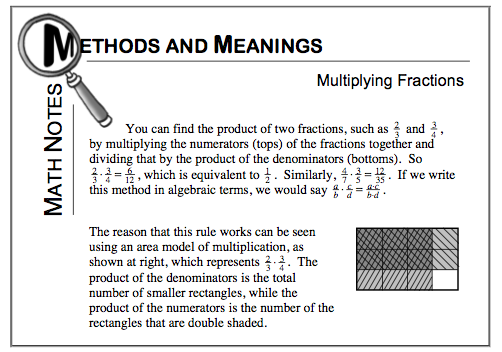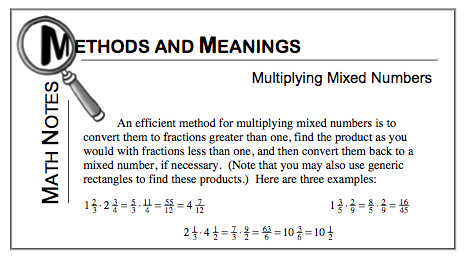### Home > MC1 > Chapter 8 > Lesson 8.2.2 > Problem8-37

8-37.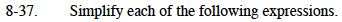For help with parts (a), (b), and (c), refer to the Math Notes boxes below.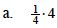$\text{It may help to look at 4 as equal to }\frac{4}{1}.$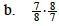The answer for part (b) is 1.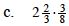You will want to convert the mixed number to a fraction before multiplying.

$\text{Can you find how many }\frac{1}{3}\text{'s are in 2?}$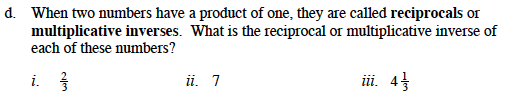For part (d), it may help to look back at part (b) of this problem.
Do you notice a way to apply that expression and solution to this question?

It may also help to turn parts (ii) and (iii) into fractions.

$\text{ If 7 equals }\frac{7}{1} \text{ and }4\frac{1}{3} \text{ equals }\frac{13}{3},\text{ can you find their reciprocals?}$

$\text{The reciprocal of (i) is }\frac{3}{2} \text{ and the answer to (iii) is }\frac{3}{13}.$

Did you find the answer to (ii)?